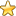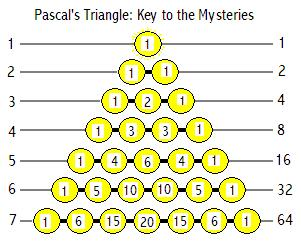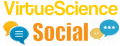Esoteric Wisdom Here are my articles on the Kabbalah, Sacred Geometry and other esoteric subjects. This section needs work and may seem unclear in its present state. Those with a deep curiosity about universal matters should persevere here because there are some very interesting ideas, some of which I have not seen anywhere else.
You are here: HomeEsoteric Wisdom# Pascal's TriangleThe laws of mathematics are eternal, universal and immutable. They are beautiful and meaningful.

By focusing our attention on the Universal Laws we atune with the Transcendent.

Number then is at the foundation of the mysteries. Pascal's Triangle displays the structure and interrelationships of Number.

Partition theory is a fundamental area of number theory. It is concerned with the number of ways that a whole number can be partitioned into whole number parts.

Five for example can be partitioned in 7 ways thus:
11111, 2111, 221, 311, 32, 41, 5.
The permutations of these 7 partitions add up to 16 thus:

11111=1 permutation
2111=4 permutations
221=3 permutations
311=3 permutations
32=2 permutations
41=2 permutations
5=1 permutation

The permutations group together naturally by their number of partitions:
4 partitions=11111=1 permutation
3 partitions=2111=4 permutations
2 partitions=221 and 311=6 permutations
1 partition=32 and 41=4 permutations
0 partitions=5=1 permutation
=1+4+6+4+1=16 permutations

So there is a relationship between the 5th level of the triangle and the number of ways that "5" can be partitioned. This relationship exists between every number and its corresponding level of Pascal's Triangle.

Note that the total number of permutations at each level is always a power of 2 ie 1, 2, 4, 8, 16, 32, 64 etc. Could it be possible that there is a relationship between the levels of the triangle/the number of ways a number can be partitioned and the 16 Geomantic Figures and the 64 Hexagrams of the I Ching etc?

Yes! There is a direct link. We take each partition as a change between yin and yang. So permutation(1,3,1) corresponds with (yin,yang,yang,yang,yin) and (yang,yin,yin,yin,yang).

Now as we are dealing with alternate yin/yang partitioning rather than simple partitioning the permutational total for a number/level is doubled.

For example: the 32 permutations of level "6" are doubled: one set for yin and one set for yang so we arrive at 64-the hexagrams are figures corresponding with every way "6" can be symmetrically/assymmetrically divided or partitioned.

So now we have established a link between abstract number theory and the "life situations" etc concepts of the I Ching. Such concepts will be seen to possess the beauty, symmetry and completeness of Number when the missunderstandings obscuring th modern view of the I Ching are removed-but that will be discussed elsewhere on this site.

# The Relationship with the Platonic Solids

The tetrahedon has 1 "outer sphere",4 corners, 6 edges and 4 faces and 1 centre.

This corresponds exactly with the 5th level of Pascal's Triangle:1,4,6,4,1.This indicates a direct relationship between the tetrahedon and the 16 Geomatic figures!

The dodecahedon has 1 "outer sphere",20 corners, 30 edges and 12 faces and 1 centre.

This seems to correspond with the 6th level of Pascal's Triangle1,6,15,20,15,6,1. This indicates a direct relationship between the dodecahedron and the 64 Hexagrams!

The cube seems to be a special case and is discussed elsewhere on this site.

You are here: HomeEsoteric WisdomCheck out the latest Esoteric Wisdom Forum Topics:Hi, I am James Barton an independent truth seeker.
VirtueScience is a unique philosophy and set of tools which I have developed over many years. It combines deep new insights with ancient wisdom.Established 2002